# can someone help me answer these 5 questions and figire this graph out please? Acid-Base Titration...

can someone help me answer these 5 questions and figire this graph out please?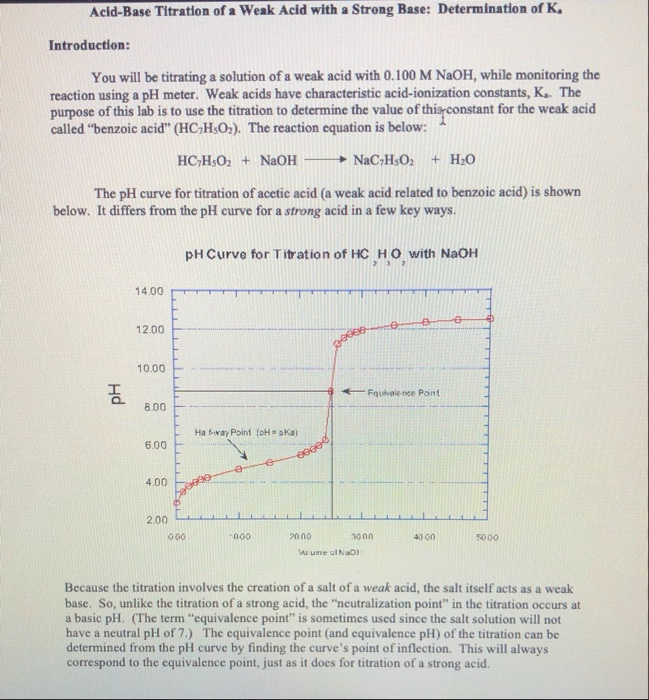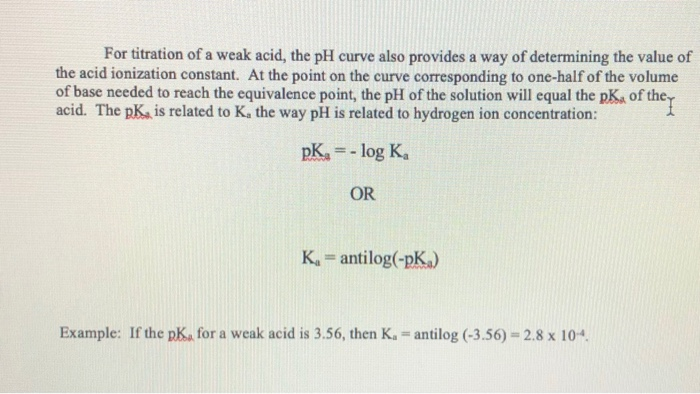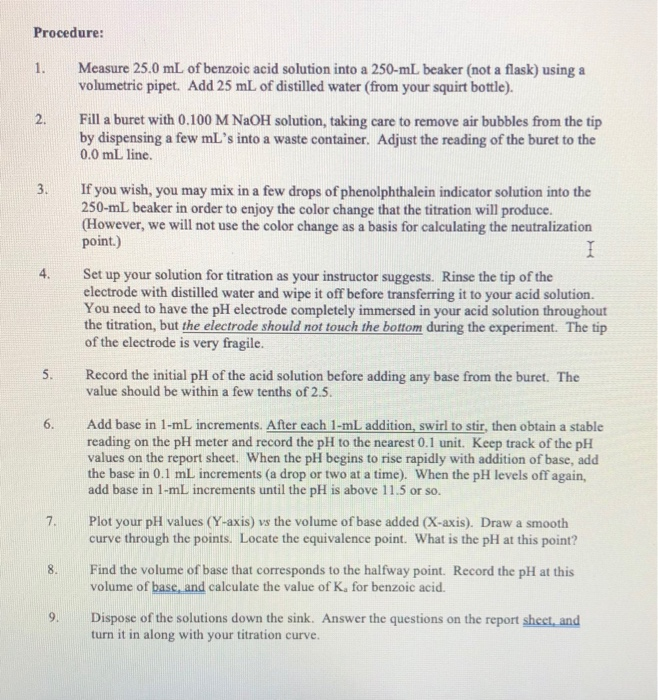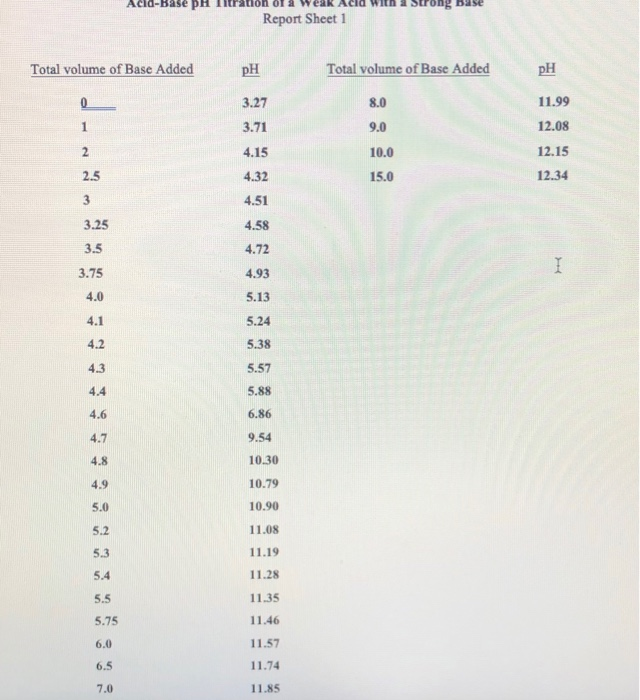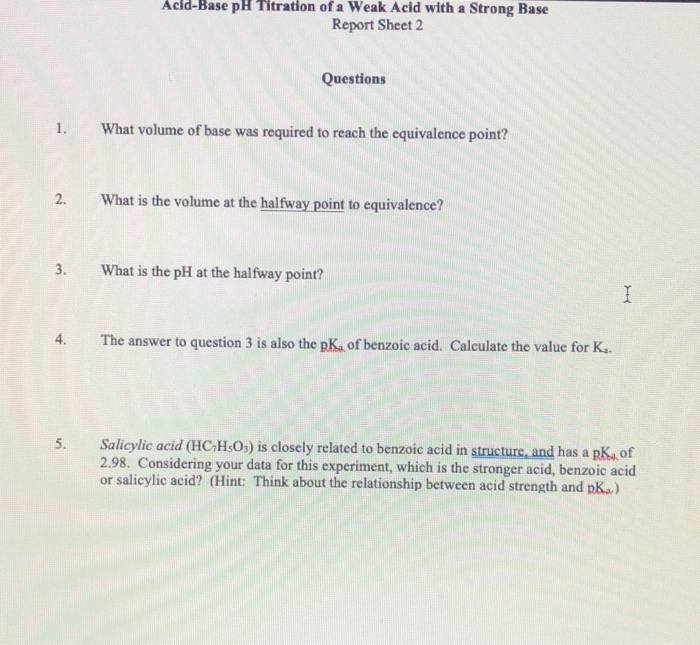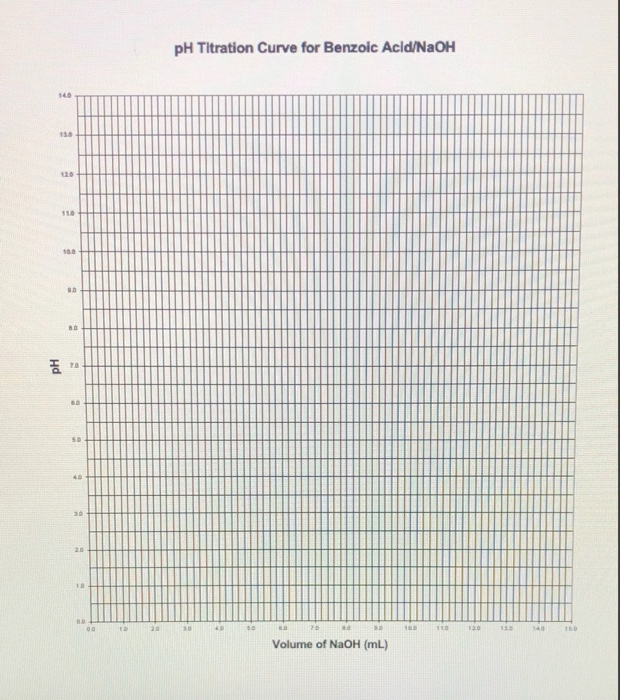Acid-Base Titration of a Weak Acid with a Strong Base: Determination of K. Introduction: You will be titrating a solution of a weak acid with 0.100 M NaOH, while monitoring the reaction using a pH meter. Weak acids have characteristic acid-ionization constants, K. The purpose of this lab is to use the titration to determine the value of this constant for the weak acid called “benzoic acid” (HC-H502). The reaction equation is below: HC,H,O2 + NaOH NaC-H502 + H2O The pH curve for titration of acetic acid (a weak acid related to benzoic acid) is shown below. It differs from the pH curve for a strong acid in a few key ways. pH Curve for Titration of HC HO with NaOH 2 3 2 14.00 12.00 ocas 10.00 рн 3Fquivalence Pont 8.00 Haway Point foH = akaj 6.00 eeee 4.00 998 2.00 000 000 40 on 5000 2000 1000 Wu une ol Nai Because the titration involves the creation of a salt of a weak acid, the salt itself acts as a weak base. So, unlike the titration of a strong acid, the "neutralization point" in the titration occurs at a basic pH. (The term "equivalence point" is sometimes used since the salt solution will not have a neutral pH of 7.) The equivalence point (and equivalence pH) of the titration can be determined from the pH curve by finding the curve's point of inflection. This will always correspond to the equivalence point, just as it does for titration of a strong acid.
For titration of a weak acid, the pH curve also provides a way of determining the value of the acid ionization constant. At the point on the curve corresponding to one-half of the volume of base needed to reach the equivalence point, the pH of the solution will equal the pKa oft of the acid. The pK, is related to K, the way pH is related to hydrogen ion concentration: pKa = -log K OR K. =antilog(-pK) Example: If the p for a weak acid is 3.56, then K. - antilog (-3.56) - 2.8 x 10-4.
Acid-Base PH Report Sheet1 Total volume of Base Added pH Total volume of Base Added pH 3.27 8.0 11.99 1 3.71 9.0 12.08 2 4.15 12.15 10.0 15.0 2.5 4.32 12.34 3 4.51 3.25 4.58 3.5 4.72 4.93 I 3.75 4.0 5.13 4.1 5.24 4.2 5.38 4.3 5.57 5.88 4.6 6.86 4.7 9.54 4.8 10.30 4.9 10.79 5.0 10.90 5.2 11.08 5.3 11.19 5.4 11.28 5.5 11.35 5.75 11.46 6.0 11.57 6.5 11.74 7.0 11.85
Acid-Base pH Titration of a Weak Acid with a Strong Base Report Sheet 2 Questions 1. What volume of base was required to reach the equivalence point? 2. What is the volume at the halfway point to equivalence? 3. What is the pH at the halfway point? I 4. The answer to question 3 is also the p. of benzoic acid. Calculate the value for K. 5. Salicylic acid (HC H:03) is closely related to benzoic acid in structure, and has a p. of 2.98. Considering your data for this experiment, which is the stronger acid, benzoic acid or salicylic acid? (Hint: Think about the relationship between acid strength and K.)
pH Titration Curve for Benzoic Acid/NaOH 140 130 100 B. pH 2 D 19 110 150 Volume of NaOH (mL)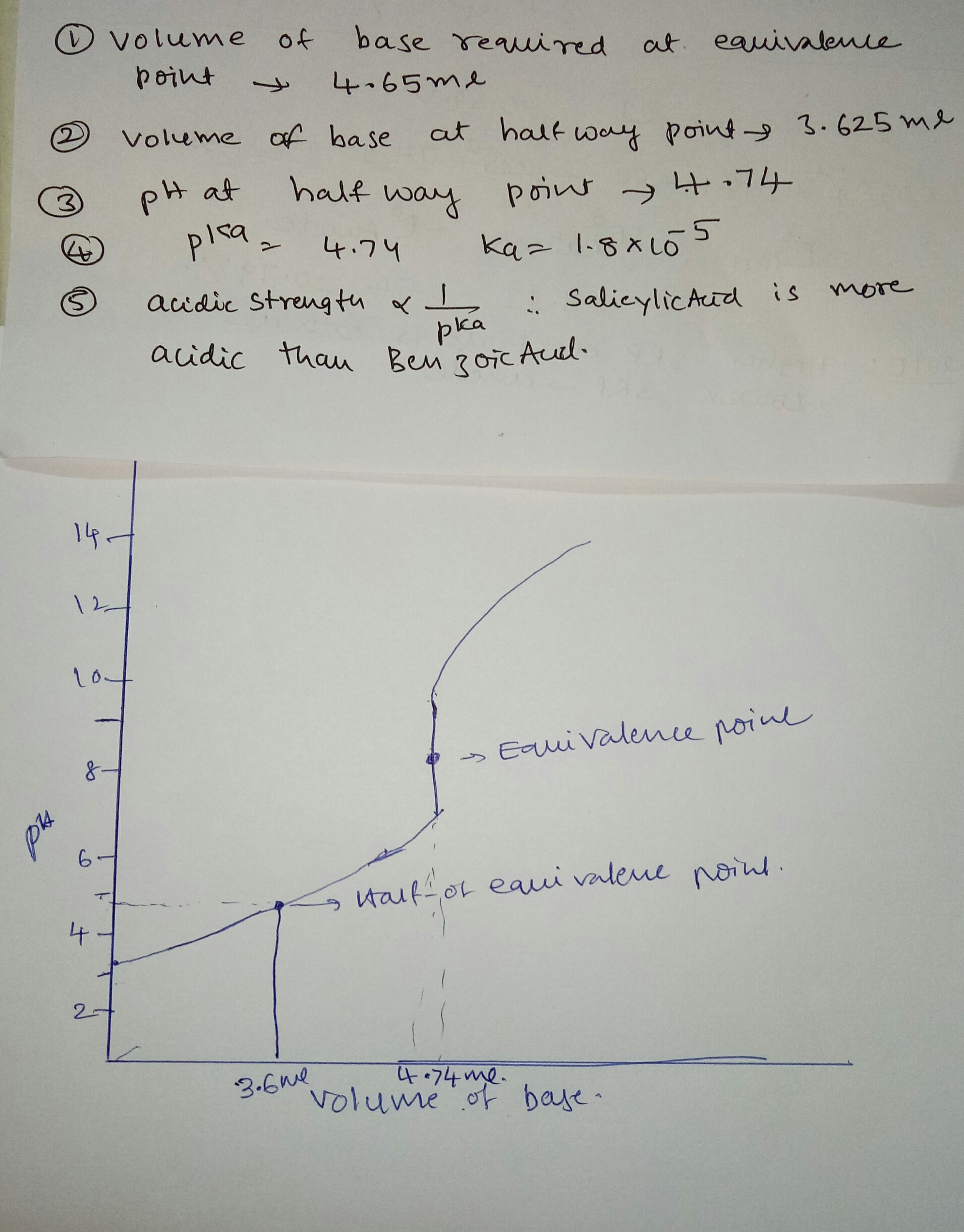#### Earn Coin

Coins can be redeemed for fabulous gifts.

Similar Homework Help Questionscan someone please help me figure out these 5 questions with the calculations shown please. and i need help with the graph ?? Acid-Base Titration of a Weak Acid with a Strong Base: Determination of K. Introduction: You will be titrating a solution of a weak acid with 0.100 M NaOH, while monitoring the reaction using a pH meter. Weak acids have characteristic acid-ionization constants, K. The purpose of this lab is to use the titration to determine the value...

• ### Answer the questions using the data and graph. please show all work. Thank you Titration of...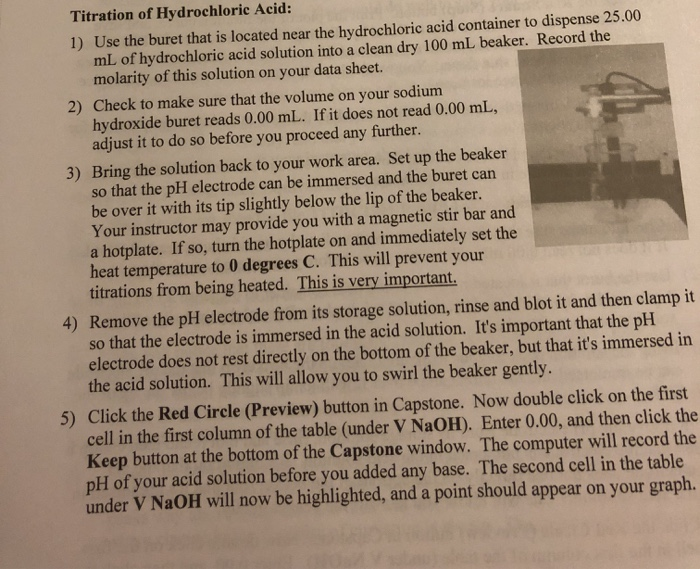Answer the questions using the data and graph. please show all work. Thank you Titration of Hydrochloric Acid: 1) Use the buret that is located near the hydrochloric acid container to dispense 25.00 mL of hydrochloric acid solution into a clean dry 100 mL beaker. Record the molarity of this solution on your data sheet. 2) Check to make sure that the volume on your sodium hydroxide buret reads 0.00 mL. If it does not read 0.00 mL, adjust it...

• ### Part A: Calculating a Theoretical Titration Curve (Weak Acid - Strong Base) Consider the titration of...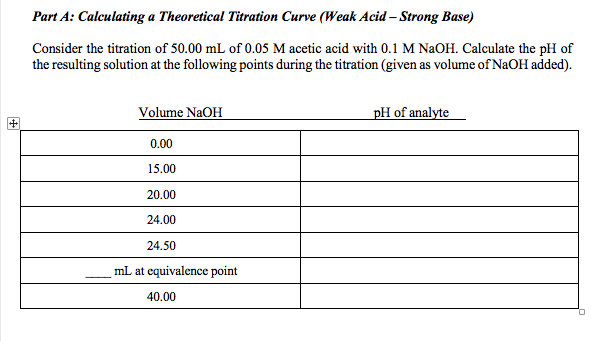Part A: Calculating a Theoretical Titration Curve (Weak Acid - Strong Base) Consider the titration of 50.00 mL of 0.05 M acetic acid with 0.1 M NaOH. Calculate the pH of the resulting solution at the following points during the titration (given as volume of NaOH added). Volume NaOH pH of analyte 0.00 15.00 20.00 24.00 24.50 mL at equivalence point 40.00

• ### Titration of weak acid with strong base pre-lab Answers can only be entered/graded between 2019-10-24 and...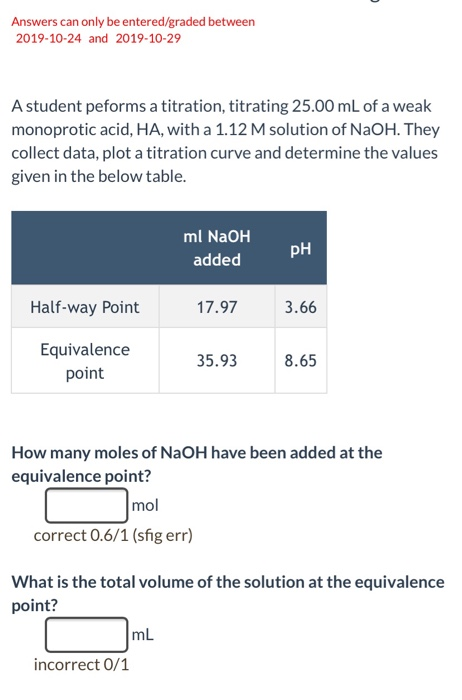Titration of weak acid with strong base pre-lab Answers can only be entered/graded between 2019-10-24 and 2019-10-29 A student peforms a titration, titrating 25.00 mL of a weak monoprotic acid, HA, with a 1.12 M solution of NaOH. They collect data, plot a titration curve and determine the values given in the below table. ml NaOH PH added Half-way Point 17.97 3.66 Equivalence point 35.938.65 How many moles of NaOH have been added at the equivalence point? r mol correct...

• ### To learn about titration types and how to calculate pH at different points of titration. In an acid-base titration, a...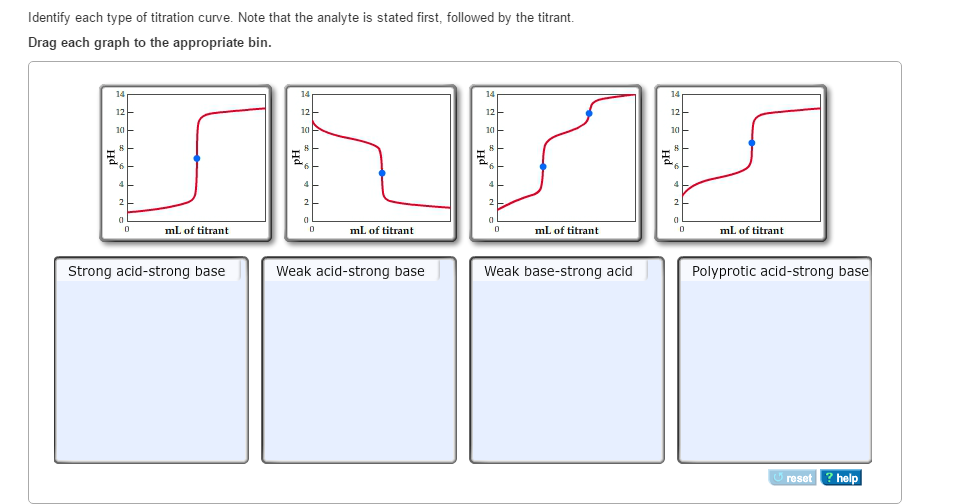To learn about titration types and how to calculate pH at different points of titration. In an acid-base titration, a titrant (solution of a base or acid) is added slowly to an analyte (solution of an acid or base). The titration is often monitored using a pH meter. A plot of pH as a function of the volume of titrant added is called a pH titration curve. Prior to the titration, the pH is determined by the concentration of the...

• ### Titration of a weak acid (CH3COOH) and strong base (NaOH), the molarity of NaOH after titration...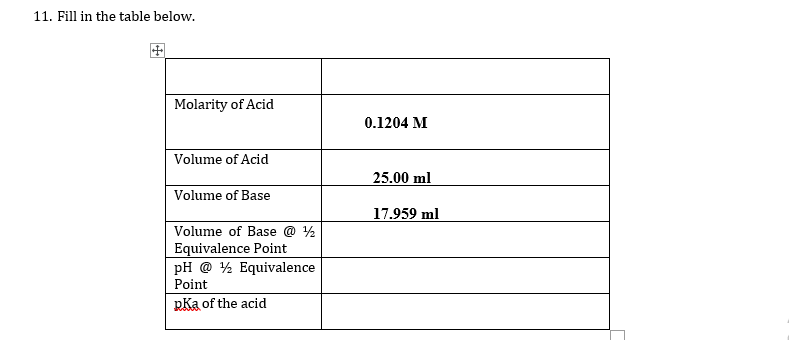Titration of a weak acid (CH3COOH) and strong base (NaOH), the molarity of NaOH after titration = 0.167 M, I need help answering to the blanks (Volume of base @ 1/2 equivalence point, pH @ 1/2 equivalence point, and pKa of the acid) on the following data table, 11. Fill in the table below. Molarity of Acid 0.1204 M Volume of Acid 25.00 ml Volume of Base 17.959 ml Volume of Base @ 12 Equivalence Point pH @ 72 Equivalence...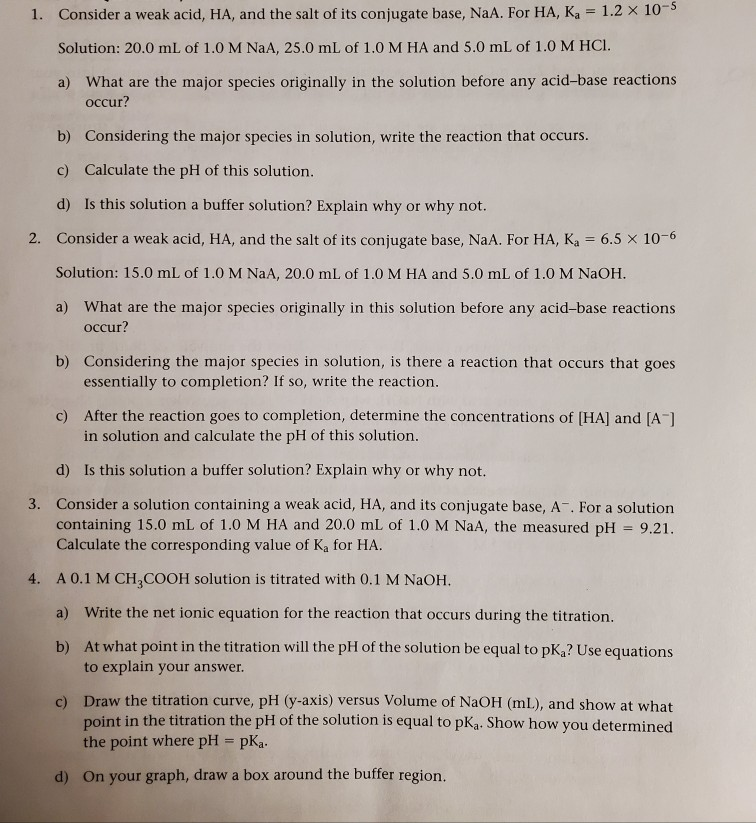can someone please help me out with questions 1-5, please To add more information this was given to me for a lab that used a weak acid and we added a strong base through titration. We just observed how the ph changes. Later we then used a buffer with a weak acid to see how buffers affect ph change. These questions are basically surrounded around those topics to help us prepare. However, I'm kinda confused about answering them because weak...

• ### Equivalence Point for Titration #1: 24.96 mL Equivalence Point for Titration #2: 25.40 mL Equivalence Point...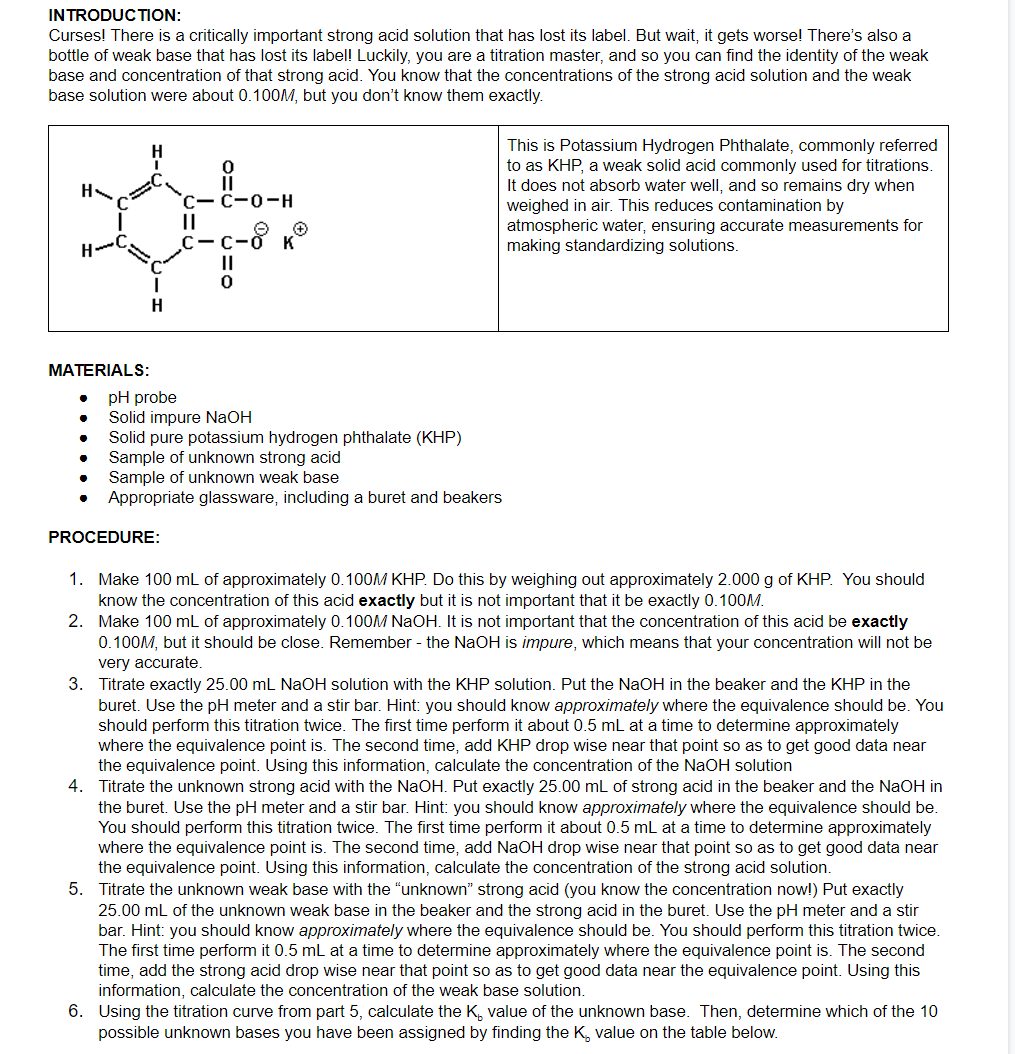Equivalence Point for Titration #1: 24.96 mL Equivalence Point for Titration #2: 25.40 mL Equivalence Point for Titration #3: 25.20 mL Midpoint pH for Titration #3: 9.80 QUESTIONS: 4) Set up the calculation required to determine the concentration of the NaOH solution via titration of a given amount of KHP. Include all numbers except the given mass of KHP. 5) Set up the calculation required to determine the concentration of the unknown strong acid via titration with a known volume...

• ### Please help me with the titration curve diagram questions. Number 22 and 23. If you could...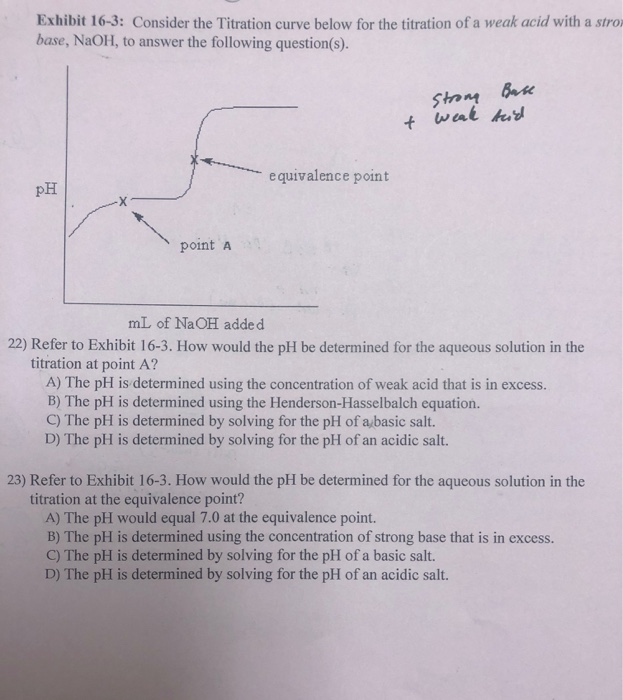Please help me with the titration curve diagram questions. Number 22 and 23. If you could also explain to me how you found the answer to the problem. Thank you! Exhibit 16-3: Consider the Titration curve below for the titration of a weak acid with a stro base, NaOH, to answer the following question(s). Strong t weak Base tid equivalence point PH point a mL of NaOH added 22) Refer to Exhibit 16-3. How would the pH be determined for...

• ### A student peforms a titration, titrating 25.00 mL of a weak monoprotic acid, HA, with a...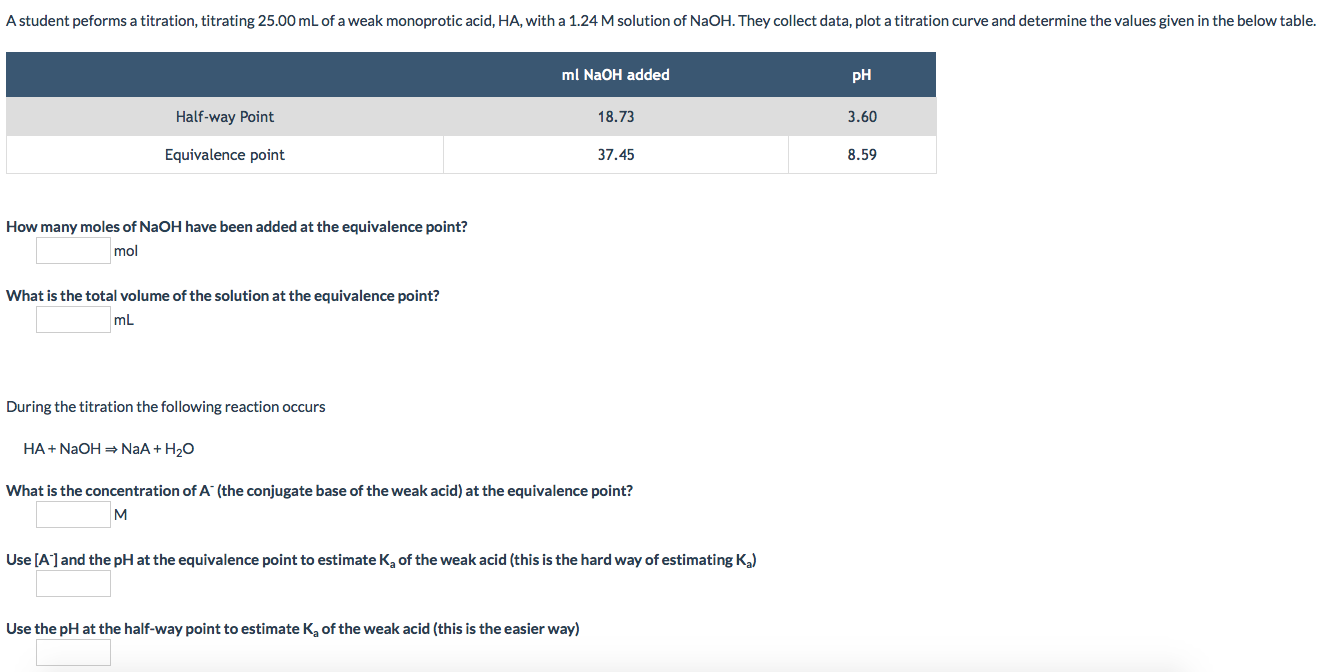A student peforms a titration, titrating 25.00 mL of a weak monoprotic acid, HA, with a 1.24 M solution of NaOH. They collect data, plot a titration curve and determine the values given in the below table. ml NaOH added pH Half-way Point 18.73 3.60 Equivalence point 37.45 8.59 How many moles of NaOH have been added at the equivalence point? mol What is the total volume of the solution at the equivalence point? mL During the titration the following...

Free Homework App